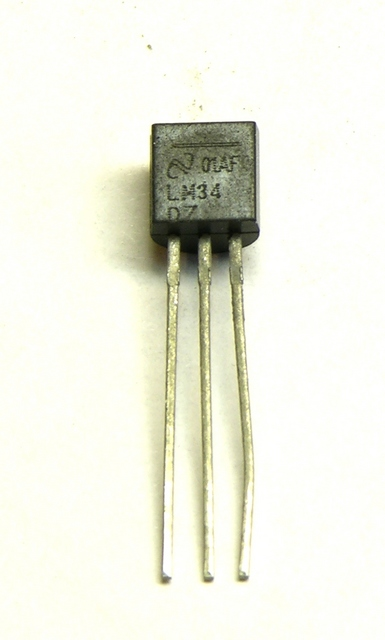## LM34DZ Fahrenheit Temperature Sensor

This started as notes I made to myself long ago for the LogoChip, but they apply equally to the Arduino A/D converters.

The LM34DZ is a temperature sensor in a TO-92 case available from All Electronics for \$2.50. It has the handy characteristic of reading an output voltage that directly corresponds to Fahrenheit temperature in a ratio of 10mV per °F. That is, at 70°F it reads 70 * 10mV = 700mV.With a direct output voltage (rather than the varying resistance that many thermal probes provide), it’s perfect for hooking to a microcontroller A/D input. So, how to convert the A/D reading back into Fahrenheit temperature?

Well, the sensor reads 10mV (or .01V) per °F. Microcontroller A/D converters tend to have 5V input and read 1024 steps over the 0-5V range.

1024 steps / 5V ≈ 205 steps / V

So

(.01V / °F) * (205 steps / V) ≈ 2 steps / °F

Thus you can get a “maybe close enough” approximation with code like

With a conversion error of +2.4%, this’ll get you within a couple of degrees at room temperature — close enough to make some macro-level observations about whether it’s getting warmer or colder for a physical computing project. Since the stated accuracy is only 1°F anyway, that’s not too bad.

If you need a more accurate conversion, you’ll need to use floating-point arithmetic if you have it (which the Arduino doesn’t [correction: does]) or find a fixed-point arithmetic library if you don’t. Or if your integer variables are large enough (at least 17 unsigned bits for temperatures up to 127°F, 18 bits up to 255°F), you can rearrange the order of calculation like so:

tempF = (1024 * analogRead(lm34Pin)) / 5;

### 9 Responses to “LM34DZ Fahrenheit Temperature Sensor”

1.Jin says:

I think Arduino does have floating point. I’ve used it, at any rate: http://www.arduino.cc/en/Reference/Float

A very simple improvement would be to feed the 3.3V output to the AREF pin for ~3.1 steps per degree. Slightly more involved would be to use an op amp to give you full scale for the temperature range of interest.

2.Keith Neufeld says:

Jim, I don’t know why I thought there was no floating point on the Arduino — you’re clearly correct. Thanks for pointing that out.

I like the LM34DZ in part because it doesn’t require external components for preamplification or level-shifting. But I really dig your idea of feeding a different voltage to AREF — thanks!

3.Dave says:

There are quite a few temperature sensor ICs in the LM family. In addition to the analog ones, there are some which are digital (e.g., National LM75/Maxim DS75). These have an I2C bus, which means that you can
put several of them on the same I2C bus (e.g., Sprinkle them around a card, enclosure, etc.).

Dave

4.Keith Neufeld says:

Dave, the I2C chips sound interesting, especially in SMT. Thanks for pointing that out!

5.bateeze henry says:

iam in uganda trying to design a LED thermometer requiring an LM34DZ temperature sensor IC but i have failed to get it. i would like alternative ICs of LM32DZ

6.bateeze henry says:

am in uganda trying to design a LED thermometer requiring an LM34DZ temperature sensor IC but i have failed to get it. i would like alternative ICs of LM34DZ.

i will greatful for your response

7.JimG says:

There’s another way to scale an a/d reading that doesn’t involve floating point math, but does require 16 bit variables.

Lets say your a/d reading is a value of 150, which is about .732 volts / 73 degrees

using a simple function such as this will allow you to scale a adc count to any value (with some limitations – see the last paragraph).

By adjusting the value of fs up or down, you can fine tune the output. fs is the value for 1024 counts.

``` uint16_t scale_adc(uint16_t adc, uint16_t fs) { uint16_t v=adc<>=1; // divide v by 2 if (fs&0x8000) { // see if fs bit 15 is set result+=v; // if so, update result } fs<<=1; // multiply fs by 2 } return result; } // a test program int main(void) { uint16_t adc,fs,temp1; adc=150; for (fs=5000; fs<=5010; fs++) { temp1=scale_adc(adc, fs); printf("fs=%d, adc=%d, scale_adc=%d\n", fs, adc, temp1); } return 0; } ```

running this gives:

```fs=5000, adc=150, scale_adc=731
```

So a value of around 5010 would be optimum for 73.2 degrees.

Going a little further, fixing fs at 5010 and varying the adc count:

```fs=5010, adc=145, scale_adc=708
8.Phew says:
9.Keith Neufeld says: